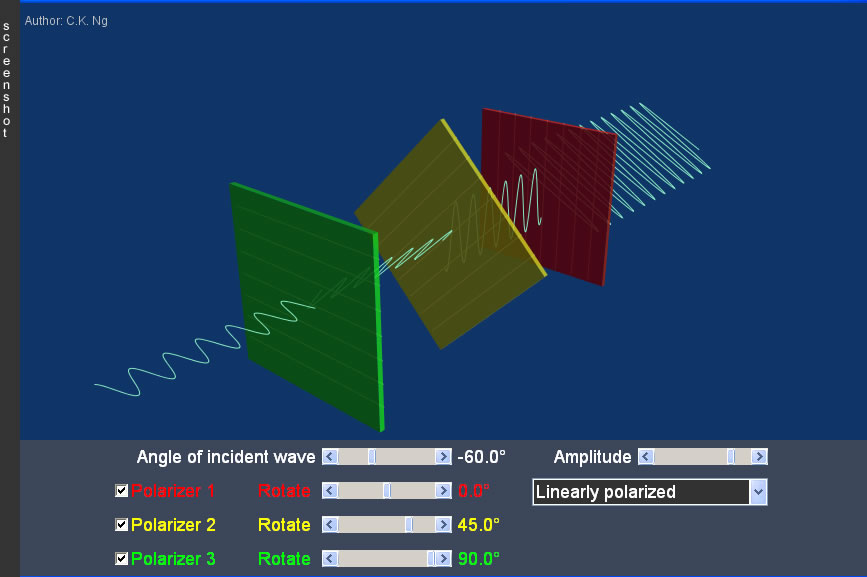Polarizer1

• The straight lines in each polarizer indicate the transmission axis of that piece.
• Polarizer is a device which transmits only the component of polariztion parallel to its transmission axis. By using a polarizer, the plane of a plane-polarized wave can be changed; a circularly polarized or unpolarized light can be changed to plane-polarized.
• When a circularly or unpolarized wave is incident on a polarizer of whatever the orientation of transmission axis, the wave intensity passing through is always one-half of the incident one (after averaging over many random changes in the case of unpolarized wave).
• No wave can pass through two polarizers whose transmission axes are crossed perpendicularly. However,when a third polarizer is inserted between them with an angle not parallel to either of them, some wave can finally pass through.
• Malus' law: The intensity (proportional to the square of amplitude) of the wave passing through a polarizer (I) is the intensity of the incident plane-polarized wave (Io) times the square of the cosine of the angle between the transmission axis of the polarizer and the incident polariztion (θ), i.e., I = Iocos2θ